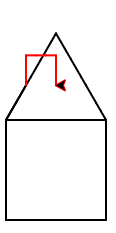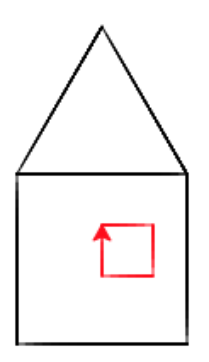# Bob Builds a House¶

Let’s combine the square and triangle code to create a drawing of a simple house.

Note

The program below has blank lines between the lines of code. The computer ignores the blank lines. We add blank lines to programs to group lines that are working together such as the lines below that draw a square. Often a comment (starts with a `#`) is used before a several lines of code to explain the purpose of the code.

Let’s imagine going on to make another partial square along the slope, to create a “chimney.”```        csp-5-5-1: Align the program pieces below to make the house and chimney figure above.  Draw the square first for the main part of the house, next draw the roof, and finally draw the chimney.from turtle import *
space = Screen()
bob = Turtle()
---
from turtle import *
space = screen()
bob = turtle() #paired
---
# Make a square
bob.forward(100)
bob.right(90)
bob.forward(100)
bob.right(90)
bob.forward(100)
bob.right(90)
bob.forward(100)
---
# Position for roof
bob.right(90)
---
# Position for roof
bob.left(90) #paired
---
# Make a roof
bob.forward(100)
bob.right(-120)
bob.forward(100)
bob.right(-120)
bob.forward(100)
bob.right(-120)
---
# Position for chimney
bob.right(-60)
bob.forward(40)
---
# Draw chimney
bob.color("red")
bob.forward(30)
bob.right(90)
bob.forward(30)
bob.right(90)
bob.forward(30)
bob.right(90)
```

Let’s make another square inside the house, to create a “window.”```        csp-5-5-2: Align the program pieces below to make the house and window figure above.  Draw the square first for the main part of the house, next draw the roof, and finally draw the window.from turtle import *
space = Screen()
bob = Turtle()
---
from turtle import *
space = screen()
bob = turtle() #paired
---
# Make a square
bob.forward(100)
bob.right(90)
bob.forward(100)
bob.right(90)
bob.forward(100)
bob.right(90)
bob.forward(100)
---
# Position for roof
bob.right(90)
---
# Position for roof
bob.left(90) #paired
---
# Make a roof
bob.forward(100)
bob.right(-120)
bob.forward(100)
bob.right(-120)
bob.forward(100)
bob.right(-120)
---
# Position for window
bob.penup()
bob.goto(50,-30)
bob.pendown()
---
# Draw window
bob.color("red")
bob.forward(30)
bob.right(90)
bob.forward(30)
bob.right(90)
bob.forward(30)
bob.right(90)
bob.forward(30)
```

Note

Discuss topics in this section with classmates.